# Multiplication principle + multiplication - math problems

#### Number of problems found: 175

• Hockey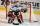Hockey match ended 8:2. How many different matches could be?
• PIN - codesHow many five-digit PIN - code can we create using the even numbers?
• MedalsIn how many ways can be divided gold, silver and bronze medal among 21 contestant?
• 2nd class combinationsFrom how many elements you can create 4560 combinations of the second class?On the menu are 12 kinds of meal. How many ways can we choose four different meals into the daily menu?
• Disco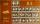On the disco goes 12 boys and 15 girls. In how many ways can we select four dancing couples?
• Subsets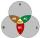How many are all subsets of set ??
• Possible combinations - word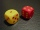How many ways can the letters F, A, I, R be arranged?
• Pins 2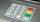how many different possible 4 digits pins can be found on the 10-digit keypad?
• DiceWe throw five times the dice. What is the probability that six fits exactly twice?
• Olympics metalsIn how many ways can be win six athletes medal positions in the Olympics? Metal color matters.
• Combinatorics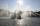The city has 7 fountains. Works only 6. How many options are there that can squirt ?
• Seven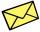Seven friends agree to send everyone a holiday card. How many postcards were sent?
• Tricolors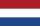From the colors - red, blue, green, black and white, create all possible tricolors.
• Two-element combinations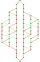Write all two-element combinations from elements a, b, c, d.
• Bits, bytes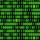Calculate how many different numbers can be encoded in 16-bit binary word?
• Tokens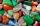In the non-transparent bags are red, white, yellow, blue tokens. We 3times pull one tokens and again returned it, write down all possibilities.
• 2nd class variationsFrom how many elements you can create 2450 variations of the second class?
• Probability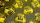What are the chances that the lottery, in which the numbers are drawn 5 of 50 you win the first prize?
• DiceWe throw 10 times a play dice, what is the probability that the six will fall exactly 4 times?

Do you have an interesting mathematical word problem that you can't solve it? Submit a math problem, and we can try to solve it.

We will send a solution to your e-mail address. Solved examples are also published here. Please enter the e-mail correctly and check whether you don't have a full mailbox.

Please do not submit problems from current active competitions such as Mathematical Olympiad, correspondence seminars etc...